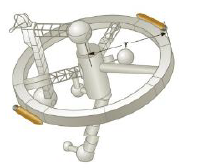Chapter 8, Problem 72P

Chapter
Section
Textbook Problem

A space station shaped like a giant wheel has a radius of 100 m and a moment of inertia of 5.00 × 108 kg · m2. A crew of 150 lives on the rim, and the station is rotating so that the crew experiences an apparent acceleration of 1g (Fig. P8.72). When 100 people move to the center of the station for a union meeting, the angular speed changes. What apparent acceleration is experienced by the managers remaining at the rim? Assume the average mass of a crew member is 65.0 kg.Figure P8.72

To determine
The apparent acceleration experienced by the managers remaining at the rim.

Explanation

Assume the total mass of crew on the rim as point particle and their moment of inertia would be defined as I=Mr2 (M=nmavg) , n is number of people live on the rim. The initial moment of inertia of the rotating system is defined as Ii=Icrew+Istation and the final moment of inertia of the system after some of crew members moved at center of the station is If=Imanagers+Istation . From conservation of angular momentum, the final angular speed of the system is Ifωf=Iiωiωf=(Ii/If)ωi and by using all these expressions, the apparent acceleration ac=rωf2 is calculated.

Given info: The radius of the giant wheel of the spaceship is 100m , the initial moment of inertia of crew in the ship is 5.00×108kgm2 , the number crew live in the rim of the ship is 150 , the apparent acceleration of the crew when they are at the rim is 1g , the number of people who moved towards the center of the rim is 100 , acceleration due to gravity is 9.80m/s2 , and the average mass of a crew member is 65.0kg .

The formula for the apparent acceleration experienced by the mangers remaining at the rim is,

ac=(150mavgr2+Istation50mavgr2+Istation)2g

• mavg is average mass of the crew member

Still sussing out bartleby?

Check out a sample textbook solution.

See a sample solution

The Solution to Your Study Problems

Bartleby provides explanations to thousands of textbook problems written by our experts, many with advanced degrees!

Get Started

Find more solutions based on key concepts